Related Articles

# How to print an entire Pandas DataFrame in Python?

• Last Updated : 26 Mar, 2021

Data visualization is the technique used to deliver insights in data using visual cues such as graphs, charts, maps, and many others. This is useful as it helps in intuitive and easy understanding of the large quantities of data and thereby make better decisions regarding it. When we use a print large number of a dataset then it truncates. In this article, we are going to see how to print the entire pandas Dataframe or Series without Truncation.

By default, the complete data frame is not printed if the length exceeds the default length, the output is truncated as shown below:

## Python3

 `import` `numpy as np``from` `sklearn.datasets ``import` `load_iris``import` `pandas as pd`` ` `# Loading irirs dataset``data ``=` `load_iris()``df ``=` `pd.DataFrame(data.data,``                  ``columns ``=` `data.feature_names)``display(df)`

Output: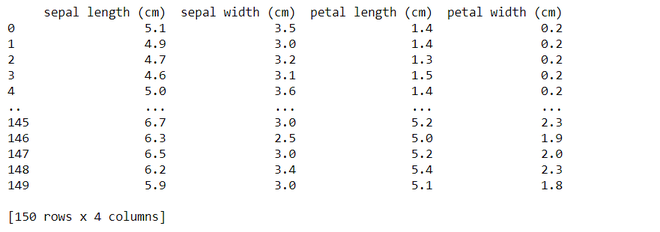### There are4 methods to Print the entire pandas Dataframe:

• Use to_string() Method
• Use pd.option_context() Method
• Use pd.set_options() Method
• Use pd.to_markdown() Method

#### Method 1: Using to_string()

While this method is simplest of all, it is not advisable for very huge datasets (in order of millions) because it converts the entire data frame into a string object but works very well for data frames for size in the order of thousands.

Syntax: DataFrame.to_string(buf=None, columns=None, col_space=None, header=True, index=True, na_rep=’NaN’, formatters=None, float_format=None, index_names=True, justify=None, max_rows=None, max_cols=None, show_dimensions=False, decimal=’.’, line_width=None)

Code:

## Python3

 `import` `numpy as np``from` `sklearn.datasets ``import` `load_iris``import` `pandas as pd`` ` `data ``=` `load_iris()``df ``=` `pd.DataFrame(data.data,``                  ``columns ``=` `data.feature_names)`` ` `# Convert the whole dataframe as a string and display``display(df.to_string())`

Output: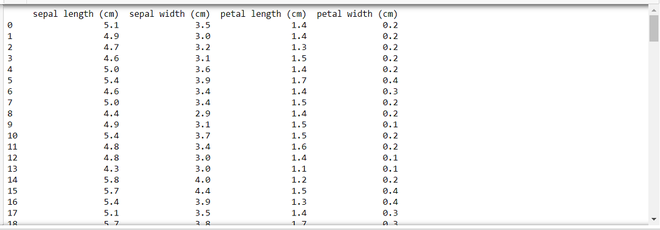#### Method 2 : Using pd.option_context()

Pandas allow changing settings via the option_context() method and set_option() methods. Both the methods are identical with one difference that later one changes the settings permanently and the former do it only within the context manager scope.

Syntax : pandas.option_context(*args)

Code:

## Python3

 `import` `numpy as np``from` `sklearn.datasets ``import` `load_iris``import` `pandas as pd`` ` `data ``=` `load_iris()``df ``=` `pd.DataFrame(data.data, ``                  ``columns ``=` `data.feature_names)`` ` `# The scope of these changes made to``# pandas settings are local to with statement.``with pd.option_context(``'display.max_rows'``, ``None``,``                       ``'display.max_columns'``, ``None``,``                       ``'display.precision'``, ``3``,``                       ``):``    ``print``(df)`

Output: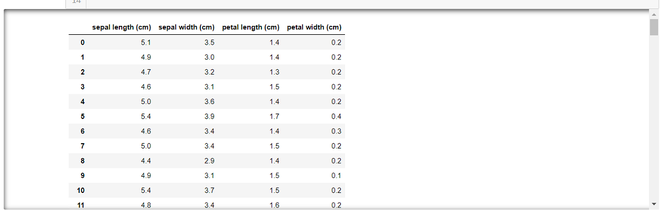Explanation:

The above code uses certain options parameters such as the ‘display.max_rows‘ its default value is 10 & if the data frame has more than 10 rows its truncates it, what we are doing is making its value to None i.e all the rows are displayed now. Similarly, ‘display.max_columns‘ has 10 as default, we are also setting it to None.

display. precision  = 3 indicates that after decimal point shows up to 3 values here all the values have 1 value and therefore it doesn’t affect this example.

#### Method 3 : Using pd.set_option()

This method is similar to pd.option_context() method and takes the same parameters as discussed for method 2, but unlike pd.option_context() its scope and effect is on the entire script i.e all the data frames settings are changed permanently

To explicitly reset the value use pd.reset_option(‘all’) method has to be used to revert the changes.

Syntax : pandas.set_option(pat, value)

Code:

## Python3

 `import` `numpy as np``from` `sklearn.datasets ``import` `load_iris``import` `pandas as pd`` ` `data ``=` `load_iris()``df ``=` `pd.DataFrame(data.data,``                  ``columns ``=` `data.feature_names)`` ` `# Permanently changes the pandas settings``pd.set_option(``'display.max_rows'``, ``None``)``pd.set_option(``'display.max_columns'``, ``None``)``pd.set_option(``'display.width'``, ``None``)``pd.set_option(``'display.max_colwidth'``, ``-``1``)`` ` `# All dataframes hereafter reflect these changes.``display(df)`` ` `print``(``'**RESET_OPTIONS**'``)`` ` `# Resets the options``pd.reset_option(``'all'``)``display(df)`

Output: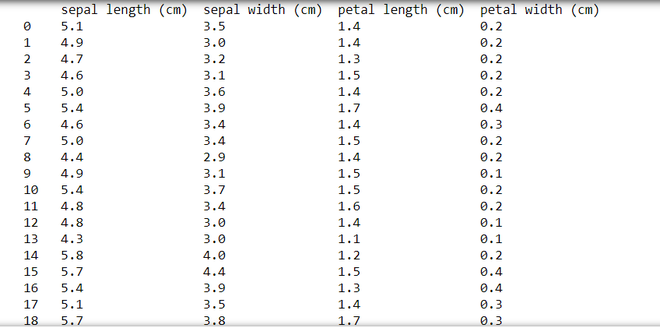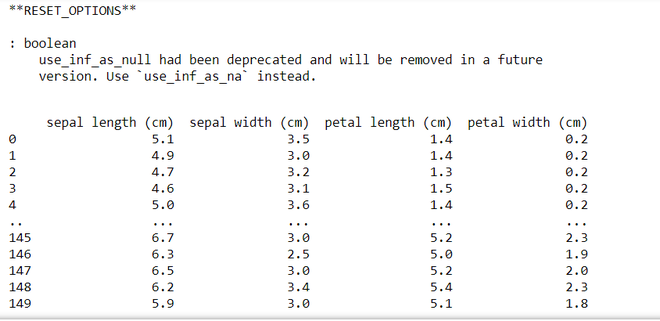#### Method 4 : Using to_markdown()

This method is similar to the to_string() method as it also converts the data frame to a string object and also adds styling & formatting to it.

Syntax : DataFrame.to_markdown(buf=None, mode=’wt’, index=True,, **kwargs)

Code:

## Python3

 `import` `numpy as np``from` `sklearn.datasets ``import` `load_iris``import` `pandas as pd`` ` `data ``=` `load_iris()``df ``=` `pd.DataFrame(data.data,``                  ``columns``=``data.feature_names)`` ` `# Converts the dataframe into str object with fromatting``print``(df.to_markdown())`

Output: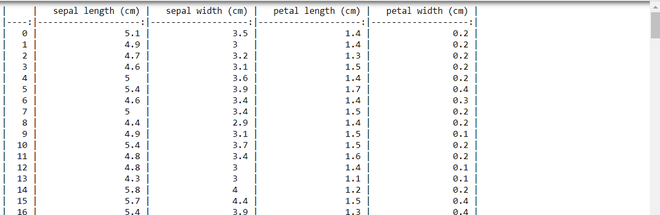Attention geek! Strengthen your foundations with the Python Programming Foundation Course and learn the basics.

To begin with, your interview preparations Enhance your Data Structures concepts with the Python DS Course. And to begin with your Machine Learning Journey, join the Machine Learning – Basic Level Course

My Personal Notes arrow_drop_up